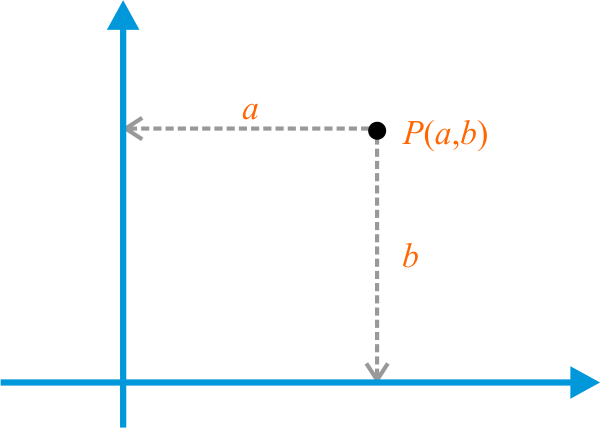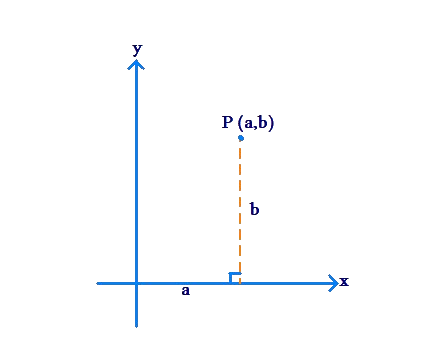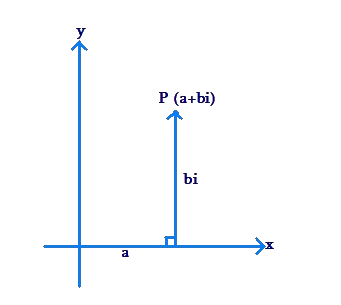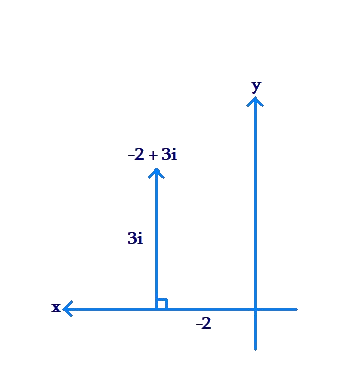# Complex Numbers – Points in the Plane

Go back to  'Complex Numbers'

## Introduction to Numbers beyond the Real Set

What is the significance of the adjective Real in the term Real Numbers? When we go deeper into Mathematics, we come across numbers that do not lie in the Real set. Does this mean that a real number is real in the sense that it exists, while a number which does not lie in the Real set is not real in the sense that it does not exist, that it is a figment of our imagination?

The answer to this question is NO.

Numbers which lie outside the Real set (specifically, Complex Numbers) are very much realor mathematically valid. In fact, the adjective Real should actually be interpreted as follows:

In a plane, any geometrical length can be described by a real number. It is in this sense that a real number is real – a real number corresponds to a “real” or physical (geometrical) length. Negative real numbers can be thought of as representing lengths to the left of 0 on a number line. The important point to understand is the association of a real number with a geometrical length.

## Complex Numbers

Let us now try to understand the basic idea of Complex Numbers (sometimes also called Imaginary Numbers) from a geometrical perspective, without going into too many details. We have seen that using the Real set, we can label every point on a line. Extending this idea, we can label every point in a plane using the Complex set. This labeling will require not one but two lines, perpendicular to one another (so we call one line the horizontal line and the other the vertical line), and crossing at the point 0, which we will call the origin. Any point in the plane can now be assigned a pair of real numbers, with the first number telling us the point’s horizontal distance from the vertical line, and the second number telling us the point’s vertical distance from the horizontal line, as shown below:In essence, the point $$P$$ can be written as $$(a,b)$$ where $$a$$ and $$b$$ will be called the coordinates of P.

In the language of complex numbers, we state that $$P$$ is the number $$a + bi$$. The fundamental fact about this representation is that the entity $$i$$ (called iota) is not a real number, in the sense that it does not lie in the Real set. On the other hand, $$a$$ and $$b$$ are real numbers. Thus, we should interpret $$a + bi$$ as a combination, or complex, of two parts (hence the name complex numbers):The real part $$a$$ tells us the point’s x-coordinate or its horizontal distance from the vertical line. The non-real part $$bi$$ tells us the point’s y-coordinate (which is $$b$$), or its vertical distance from the horizontal line. Note that b itself is real, whereas $$i$$ is not a real number, and hence $$a + bi$$ is not a real number.

✍Note: Real Numbers can be labeled by using one horizontal number line but Complex Number will require two lines perpendicular to one another, so-called x-coordinate and y-coordinate.

In our discussion on Real Numbers, we saw that every point on a straight number line can be represented using a real number, and every real number corresponds to a point on the real number line. In other words, the Real set enables us to label points on a line with unique number representations.

Is it possible to label points in a plane using numbers? In Coordinate Geometry, we have studied that this is possible using a pair of numbers: an x-coordinate and a y-coordinate. The position of any point in the plane can be uniquely specified using these two coordinates:In the language of Complex Numbers, the point .$$P\left( {a,b} \right)$$. can be represented as the number $$a + bi$$. Let us examine this entity $$a + bi$$ in more detail.

• The number $$a + bi$$ is a sum, or complex, of two entities: a and bi (hence the name complex numbers)

• The first entity, a, is a real number. It tells us the x-coordinate of the point P.

• The second entity, bi (or $$b \times i$$), is a non-real number, in the sense that it does not lie in the Real set. You should interpret bi as “b units along the i direction”, where the i direction is perpendicular to the real line.

Thus, to reach the point $$P\left( {a + bi} \right)$$, you need to move a units in the real direction (along the real line), and b units in the i direction (perpendicular to the real line):For example, let us plot the point corresponding to the number $$- 2 + 3i$$ in the plane. We move $$- 2$$ units along the real direction, and 3 units along the i-direction:Thus, every point in the plane can be represented using a number of the form $$x + iy$$. Conversely, every number of the form $$x + iy$$ corresponds to a point in the plane.

In other words, just like the Real Set enables us to label every point on the Real Line, the Complex Set enables us to label every point in the Complex Plane:

• Real Set $$\Leftrightarrow$$ Real Line

• Complex Set $$\Leftrightarrow$$ Complex Plane

## What is IOTA?

What exactly is $$i$$? Who invented $$i$$ and for what reason?

The answers to these questions can be found in the history of the evolution of Mathematics. The recognition that there are numbers which lie outside the Real set, and that they are perfectly valid mathematical entities, took centuries of effort by some of the best mathematical minds of their time. The important thing for you to remember at this point is that complex numbers are as real as real numbers are. In fact, they are even more powerful. Using the Real set, we can only label points on a line. Using the Complex set, we can label every point in a plane. This observation should also tell you that the Real set is, in fact, a subset of the Complex set, that is, every real number is a complex number; any real number $$x$$ can be written in the complex form: $$x = x+ i0$$.

✍Note: The set of real numbers is the subset of the set of complex numbers.

## Solved Example:

Example 1: Write the x-coordinate and y-coordinate of the following complex numbers?

1. $$3 + 5i$$
2. $$- 8 - 3i$$
3. $$\frac{{18 + 24i}}{6}$$

Solution: We know that any complex number is of the form $$a + bi$$.

Also, the real part $$a$$ tells us the point’s x-coordinate and non-real part $$bi$$ tells us the point’s y-coordinate (which is $$b$$).

For $$3 + 5i$$,

Clearly, $$\boxed{x - {\text{coordinate}} = 3}$$ and $$\boxed{y - {\text{coordinate}} = 5}$$

For $$- 8 - 3i$$,

This can be written as $$- 8 + ( - 3i)$$

Clearly, $$\boxed{x - {\text{coordinate}} = -8}$$ and $$\boxed{y - {\text{coordinate}} = -3}$$

For $$\frac{{18 + 24i}}{6}$$,

We can simply it as follow,

$\frac{{18 + 24i}}{6} = \frac{{18}}{6} + \frac{{24i}}{6} = 3 + 4i$

Clearly, $$\boxed{x - {\text{coordinate}} = 3}$$ and $$\boxed{y - {\text{coordinate}} = 4}$$Challenge: If the coordinates of a point in a plane are $$\left( {\frac{1}{2},9} \right)$$, represent it as a complex number.

⚡Tip: x-coordinate tells us the real part $$a$$ and y-coordinate tells us the non-real part $$bi$$.

Complex Numbers
Complex Numbers
Complex Numbers
grade 10 | Questions Set 2
Complex Numbers
grade 10 | Questions Set 1

More Important Topics
Numbers
Algebra
Geometry
Measurement
Money
Data
Trigonometry
Calculus